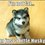# Help needed!

How to find the last two digits of $3^{17}$?Note by Anik Mandal
5 years, 8 months ago

This discussion board is a place to discuss our Daily Challenges and the math and science related to those challenges. Explanations are more than just a solution — they should explain the steps and thinking strategies that you used to obtain the solution. Comments should further the discussion of math and science.

When posting on Brilliant:

• Use the emojis to react to an explanation, whether you're congratulating a job well done , or just really confused .
• Ask specific questions about the challenge or the steps in somebody's explanation. Well-posed questions can add a lot to the discussion, but posting "I don't understand!" doesn't help anyone.
• Try to contribute something new to the discussion, whether it is an extension, generalization or other idea related to the challenge.

MarkdownAppears as
*italics* or _italics_ italics
**bold** or __bold__ bold
- bulleted- list
• bulleted
• list
1. numbered2. list
1. numbered
2. list
Note: you must add a full line of space before and after lists for them to show up correctly
paragraph 1paragraph 2

paragraph 1

paragraph 2

[example link](https://brilliant.org)example link
> This is a quote
This is a quote
    # I indented these lines
# 4 spaces, and now they show
# up as a code block.

print "hello world"
# I indented these lines
# 4 spaces, and now they show
# up as a code block.

print "hello world"
MathAppears as
Remember to wrap math in $$ ... $$ or $ ... $ to ensure proper formatting.
2 \times 3 $2 \times 3$
2^{34} $2^{34}$
a_{i-1} $a_{i-1}$
\frac{2}{3} $\frac{2}{3}$
\sqrt{2} $\sqrt{2}$
\sum_{i=1}^3 $\sum_{i=1}^3$
\sin \theta $\sin \theta$
\boxed{123} $\boxed{123}$

Sort by:

Last two digits of a number is the remainder obtained on dividing that number by 100

$3^{17}=3.9^{8}=3.(10-1)^{8}$

In The expansion of $(10-1)^{8}$ all terms are divisible by 100 except two terms which are

$^{8}C_{7}.10.(-1)^{7}+(-1)^{8}=-79$ Remember the 3 which we had separated before, multiply it by - 79 to get - 237

On dividing - 237 by 100 we get the remainder as 63

- 5 years, 8 months ago

Thanks bro!

- 5 years, 8 months ago

Very easy problem bro :P

Hint : Find ${3}^{17} \mod 100$.

- 5 years, 8 months ago

Yes i know. But how to compute it?

- 5 years, 8 months ago

Hmm.. answer comes $63$. Is that correct? ( I wasted $2$ pages of my copy to discover that ${ 3 }^{ 15 }\equiv 7 \mod 100$ :P)

- 5 years, 8 months ago

Yes correct! How you got that?

- 5 years, 8 months ago

If you calculate 3^17,you will get the answer,129140163,and to extract its 2 digits from right,mod it by 100 that is, number%100.Then you would get the answer 63.

- 5 years, 8 months ago

Suppose it is a big number.Then you can't calculate it..in this case you can

- 5 years, 8 months ago